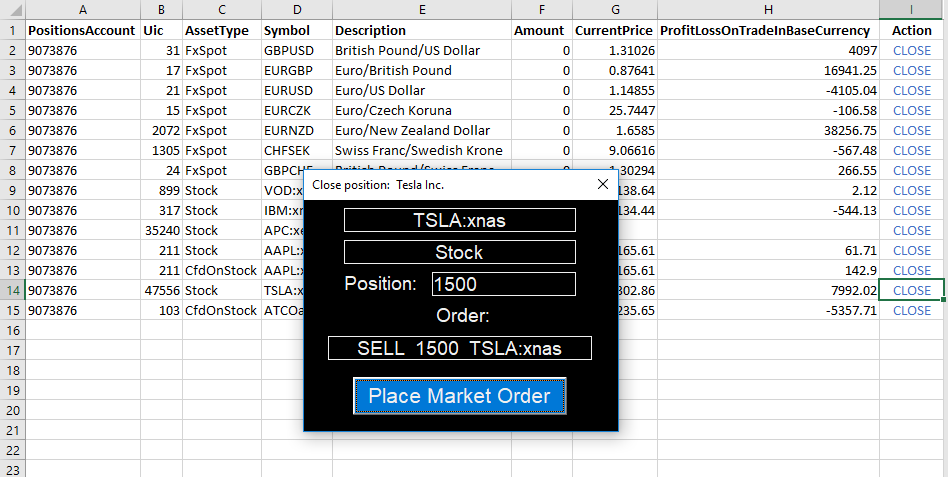OpenAPI for Excel

With the trade ticket functionality developed in Tutorial 7, only a couple of modifications are required to build a tool that closes positions with a prompt similar to the one used before. In this case, the requirements for this functionality are fairly straightforward:

1. The NetPositions tab keeps track of all of the client's positions using a subscription setup.
2. For each position, a CLOSE action is provided, which opens the trade prompt.
3. The prompt loads all relevant data and calculates the amount Buy/Sell required to close the position.
4. After the user confirms, the OpenApiClosePosition() formula is called to send a trade order to the OpenAPI.
5. If a position is already closed (Amount = 0), a warning message is shown.

The final result looks as below:## VBA solution

As before, the UserForm object in VBA is used to display an interactive prompt. On the NetPositions tab, a similar tracking mechanism is used to check whether the user is clicking a cell marked CLOSE (see below). Key points in this script are the same as before, with the addition of the following:

• The tradeamount variable is calculated based on the client's current position. Long positions are translated into a Sell order with the same (positive) amount, whereas short positions (which are negative) are translated to a Buy order with the corresponding (positive) amount.
• If a position is already closed, the entire subroutine is skipped and a message is displayed informing the user.
• As before, the Close_Ticket is loaded in the center of the screen, with all required parameters pre-loaded.
```Private Sub Worksheet_SelectionChange(ByVal Target As Range)
Dim uic As Long
Dim assettype As String
Dim desc As String
Dim symbol As String
Dim dir As String
Dim amount As Long
Dim posid As Double
Dim accid As String
Dim acckey As String

If Target.CountLarge = 1 Then 'if the selection is a single cell

If Target.Text = "CLOSE" Then 'when CLOSE is clicked
uic = Target.Offset(0, -8).Value  'assign UIC
assettype = Target.Offset(0, -7).Value  'assign AssetType
symbol = Target.Offset(0, -6).Value 'assign Symbol
desc = Target.Offset(0, -5).Value 'assign description
amount = Target.Offset(0, -4).Value 'assign amount value
posid = Target.Offset(0, -9).Value 'assign amount value
accid = Target.Offset(0, -10).Value 'assign accountid

If amount > 0 Then
dir = "SELL"
Else
End If

With Close_Ticket 'launch close ticket
'load close ticket in the center of the Excel window
.StartUpPosition = 0
.Left = Application.Left + (0.5 * Application.Width) - (0.5 * .Width)
.Top = Application.Top + (0.5 * Application.Height) - (0.5 * .Height)

.Caption = "Close position:  " + desc
.symbollabel.Caption = symbol
.assettypelabel = assettype
.positionamount.Caption = amount
.id.Caption = posid
.ordertext.Caption = dir + "  " + CStr(tradeamount) + "  " + symbol
.account.Caption = accid
End With

'display ticket
Close_Ticket.Show

End If

End If

End Sub```

The Close_Ticket itself only includes a single subroutine linked to the Place Market Order button. This button performs the exact same steps as in the previous tutorial, except for:

• The direction of the order is taken from theordertext field, which in turn is pre-loaded when the ticket is launched.
• The tradeamount is derived using the client's current position (similar to the above).
• OpenApiClosePosition() ia called instead of PlaceOrder().

This way, the user is able to quickly open and close positions through the Excel spreadsheet. In addition, all current positions are updated in real-time, as are the trade messages (to keep track of trade confirmations).

```Private Sub placeorder_Click()

acckey = Application.VLookup(account.Caption, Sheet3.Range("A3:B7"), 2, False)
uic = ActiveCell.Offset(, -8)

ordertext.Caption = "Sending order.."

If ActiveCell.Offset(, -4) < 0 Then
Else
dir = "Sell"
End If

body = "{" & _
"'Orders':[{" & _
"'AccountKey':'" & [AccountKey] & "', " & _
"'Amount':" & tradeamount & ", " & _
"'AssetType':'" & assettypelabel.Caption & "', " & _
"'BuySell':'" & dir & "', " & _
"'Uic':" & uic & ", " & _
"'OrderType':'Market', " & _
"'OrderDuration':{'DurationType':'DayOrder'}, " & _
"'ManualOrder':true}], " & _
"'PositionId':" & ActiveCell.Offset(, -9) & _
"}"